# Some Important Characteristics of a Wave

A wave is a sort of disturbance which originates from some vibrating source and travels outward as a continuous sequence of alternating crests and troughs. Every wave has five important characteristics, namely, wavelength , frequency , velocity (c), wave number and amplitude (a).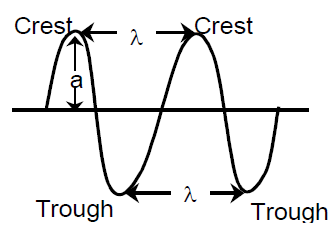Ordinary light rays, X–rays, α–rays, etc. are called electromagnetic radiations because similar waves can be produced by moving a charged body in a magnetic field or a magnet in an electric field.

These radiations have wave characteristics and do not require any medium for their propagation.

i) Wave length (λ): The distance between two neighbouring troughs or crests is known as wavelength. It is denoted by λ and is expressed in cm, m, nanometers (1nm=10^–9m) or Angstrom (1Å=10^–10m).

ii) Frequency (υ): The frequency of a wave is the number of times a wave passes through a given point in a medium in one second. It is denoted by υ (nu) and is expressed in cycles per second (cps) or hertz (Hz) 1Hz = 1cps.

The frequency of a wave is inversely proportional to its wave length (λ)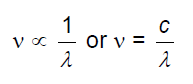iii) Velocity: The distance travelled by the wave in one second is called its velocity. It is denoted by c and is expressed in cm/ sec.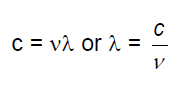iv) Wave number: It is defined as number of wavelengths per cm.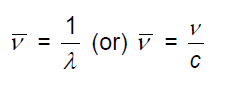v) Amplitude: It is the height of the crest or depth of the trough of a wave and is denoted by a. It determines the intensity or brightness of the beam of light.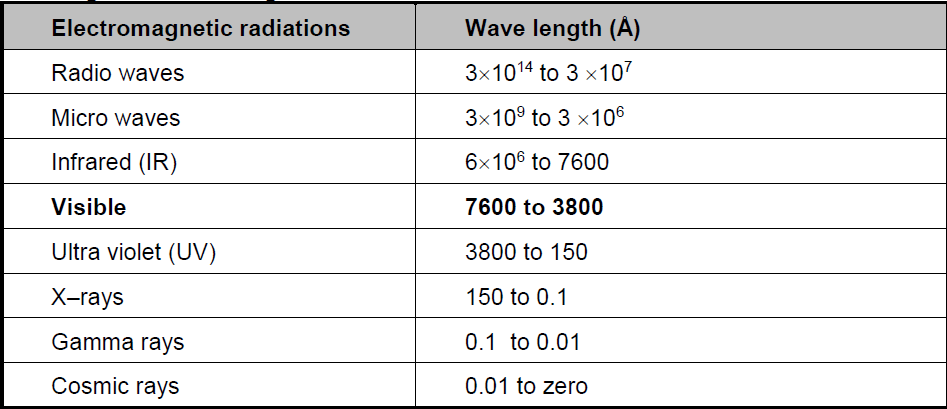CBSE Class 11 Chemistry